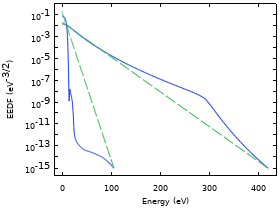# Application Gallery

## DC Glow Discharge Coupled with the Two-Term Boltzmann Equation

Application ID: 73091

This tutorial models a DC glow discharge by solving plasma fluid type equations fully coupled with the homogeneous and time-independent electron Boltzmann equation in the classical two-term approximation. The approximated Boltzmann equation is solved for each position of space and is coupled with the fluid type equations by way of the electron mean energy. The rate constants of electron impact reactions and the electron transport parameters are obtained by suitable integration of the computed electron energy distribution function over electron scattering cross sections.This model example illustrates applications of this type that would nominally be built using the following products: